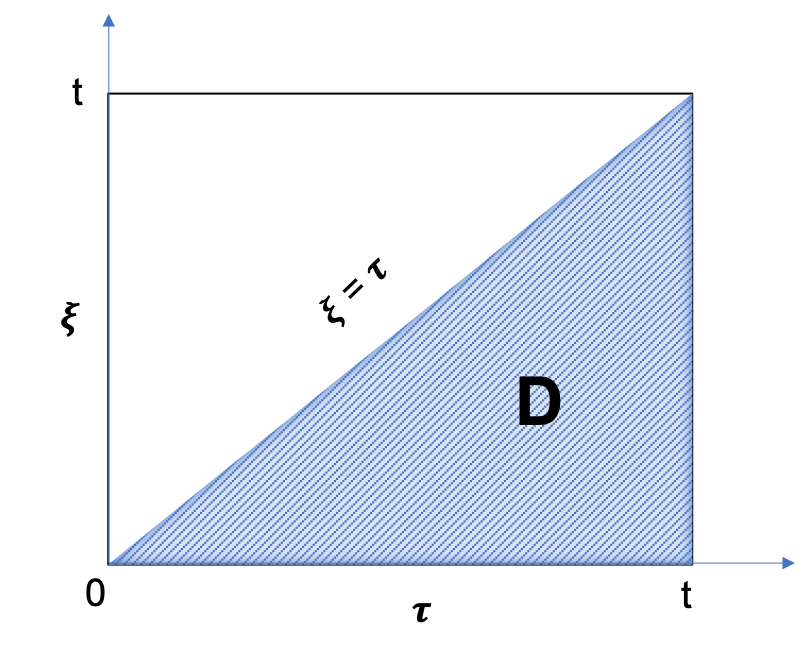# Derivation of Radial VEH Aquifer Drive @model

Consider a system of net hydrocarbon pay and finite or infinite volume Aquifer as a radial composite reservoir with inner composite area being a Net Pay Area and outer composite area an Aquifer

(see schematic and notations on Fig. 1 at Radial VEH Aquifer Drive @model).

The Aquifer's outer boundary may be "no-flow" for finite-volume Aquifer or constant pressure for infinite-volume Aquifer.

The transient pressure diffusion in the outer (Aquifer) composite area is going to honour the following equation:

Driving equationInitial condition

Homogeneous Aquifer reservoir with $//$

Initial Aquifer pressure is considered to be the same as Net Pay Area
 (1)
 (2)

Inner Aquifer boundaryOuter Aquifer boundary is one of the two below:
Pressure variation at the contact with Net Pay Are"No-flow" "Constant pressure"
 (3)
 (4)
 (5)

Consider dimensionless solution $//$ of the following equation:

 (6)
 (7)

 (8)
 (9)

which represents a unique function of dimensionless time $//$ and distance $//$.

Now consider a convolution integral:

 (10)
 (11)

One can easily check that (10) honours the whole set of equations (1)(4)/(5) and as such defines a unique solution of the above problem.

Now having the pressure dynamics in hand one can calculate the water influx.

The water flowrate within $//$ sector angle at the interface with net pay is:

 (12)

where $//$ is flow velocity at Net Pay ↔ Aquifer contact boundary, which is:

 (13)

where $//$ is Aquifer mobility.

The water flowrate is going to be:

 (14)

and cumulative water flux is going to be:

 (15)

Substituting (10) into (15) leads to:

 (16)
 (17)
 (18)

The above integral represents the integration over the $//$ area in $//$ plane (see Fig. 1):

 (19)Fig. 1. Illustration of the integration $//$ area in $//$ plane

Changing the integration order from $//$ to $//$ leads to:

 (20)

Replacing the variable:

 (21)

and cumulative influx becomes:

 (22)

where $//$ is water influx constant and which leads to:

 (23)

where

 (24)

is a unique dimensionless function of dimensionless time $//$ which can be tabulated via numerical solution or approximated by closed-form expression.